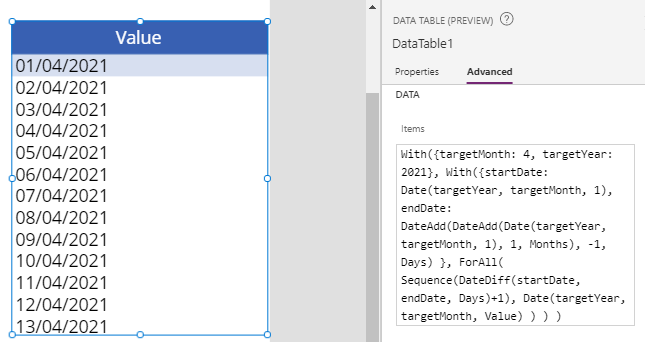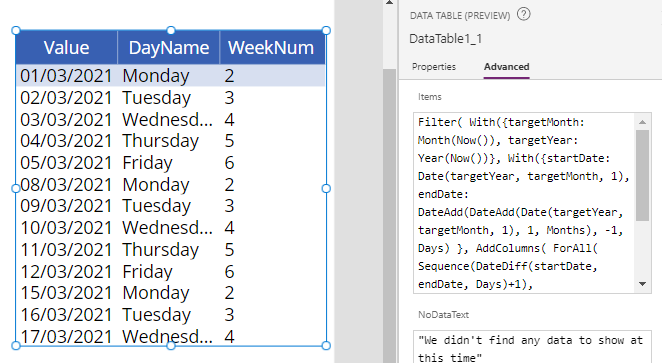# Formulas - how to return all days or working days in a month

They can often be the requirement to return all working days in a month, particularly in cases where we develop timesheet or reporting type apps. This post describes the formula to carry out this task.

There are often scenarios where we need to return all working days in a month.

Typical use case scenarios usually involve timesheet or reporting type apps. There may be a requirement to populate drop down boxes with working days only, or to build collections that are 'keyed' by working dates.

This post covers this topic by highlighting common tasks that include:
• how to return all days in a given month
• how to return all days in the current month
• how to return all working days in a month
• how to return weekends in the month
• how to include additional details in the output, such as day names

## Basic formula to return all days in a month

Let's begin by looking at the basic formula that returns all days in a month. We'll use this as the basis to return all working days as we progress. The key input values here are the targetMonth and targetYear values. We replace these with the month and year of our desired output.

``With({targetMonth: 4, targetYear: 2021},     With({startDate: Date(targetYear, targetMonth, 1),            endDate: DateAdd(DateAdd(Date(targetYear, targetMonth, 1), 1, Months), -1, Days)          },     ForAll(             Sequence(DateDiff(startDate, endDate, Days)+1),              Date(targetYear, targetMonth, Value)            )    ))``

The screenshot beneath shows the output when we apply this formula to the items property of a data table. In practice, a typical use case scenario would be to apply this formula to the items property of a drop down control.To provide additonal details on how this formula works, the inputs to this formula are targetMonth and targetYear. The second 'with' block derives the start and end dates for the given month and year. In this case, this part of the formula derives a startDate of '2021-04-01', and an endDate of '2021-04-30'

The ForAll part of the formula produces the output. It loops over a sequence of numbers from 1 to 30. We produce this sequence by calling the Sequence function, and passing the number of days between startDate and endDate, through the help of the DateDiff function. Because the DateDiff function calculates the date difference that is exclusive of the end date, we add 1 day to give our desired output of 30 days.

The sequence function returns a single column table with the column name 'value'. For each iteration of the ForAll loop, we return an output date with a day that corresponds to the sequence value (eg, 1 to 30).

## Formula to return all days in the current month

The above formula returns all days for the hardcoded month April 2021. The variation of this formula beneath calls the Now() function to return all days for the current month and year.

``With({targetMonth: Month(Now()), targetYear: ``Year(Now())},     With({startDate: Date(targetYear, targetMonth, 1),            endDate: DateAdd(DateAdd(Date(targetYear, targetMonth, 1), 1, Months), -1, Days)          },     ForAll(             Sequence(DateDiff(startDate, endDate, Days)+1),              Date(targetYear, targetMonth, Value)            )    ))``

## Formula to return all working days in the current month

To adapt the formula so that it returns only working days (eg, Monday to Friday), we filter the output to return only days that match a weekday value between 2 and 6. The weekday function returns a weekday number where 1 equals Sunday, and 7 equals Saturday.

``Filter(    With({targetMonth: Month(Now()), targetYear: Year(Now())},        With({startDate: Date(targetYear, targetMonth, 1),             endDate: DateAdd(DateAdd(Date(targetYear, targetMonth, 1), 1, Months), -1, Days)            },        ForAll(                Sequence(DateDiff(startDate, endDate, Days)+1),                 Date(targetYear, targetMonth, Value)                )        )    ),    Weekday(Value) > 1 And Weekday(Value) < 7 )``

## Formula to return all weekends in the current month

To return only weekends (Saturdays and Sundays) for the current month, we adapt the formula to return only records that match the weekday values 1 or 7.

``Filter(    With({targetMonth: Month(Now()), targetYear: Year(Now())},        With({startDate: Date(targetYear, targetMonth, 1),             endDate: DateAdd(DateAdd(Date(targetYear, targetMonth, 1), 1, Months), -1, Days)            },        ForAll(                Sequence(DateDiff(startDate, endDate, Days)+1),                 Date(targetYear, targetMonth, Value)                )        )    ),    Weekday(Value) = 1 Or Weekday(Value) = 7 )``

## Formula to return all working days (including day names) in the current month

Finally we can extend our output to include additional, custom columns. We accomplish this by calling the AddColumns function.

The following example highlights how to add the day name (eg, Monday, Tuesday, Wednesday etc), and the weekday number.

``Filter(    With({targetMonth: Month(Now()), targetYear: Year(Now())},        With({startDate: Date(targetYear, targetMonth, 1),             endDate: DateAdd(DateAdd(Date(targetYear, targetMonth, 1), 1, Months), -1, Days)            },        AddColumns(             ForAll(                Sequence(DateDiff(startDate, endDate, Days)+1),                 Date(targetYear, targetMonth, Value)                )                , "DayName", Text(Value, "dddd")                , "WeekNum", Weekday(Value))        )    ),    Weekday(Value) > 1 And Weekday(Value) < 7 )``

For additional help, the post here describes the formula to produce day names.

The screenshot beneath illustrates the output of this formula.## Conclusion

They is often the requirement to return specific days for a month, including working days or weekends. This post described the formula that enables us to carry out this task.